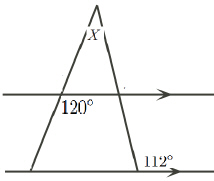Name:    MC Angles and Lines

Multiple Choice
Identify the choice that best completes the statement or answers the question.

1.

In a right angled triangle, if one angle is 55 degrees, what is the measure of the smallest angle in this triangle?
 a. 25 degrees c. 35 degrees b. 45 degrees d. 90 degrees

2.

If two straight lines intersect as shown, then what is the value of “x - y”?a. 40 c. 80 b. 60 d. 100

3.

Given the following rectangle, what is the value of “y”?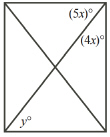a. 30 d. 60 b. 40 e. 45 c. 50 f. 55

4.

What is the value of “x”?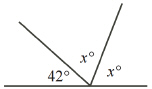a. 138 c. 59 b. 79 d. 69

Numeric Response

5.

Given that the triangle is an isosceles triangle, what is the angle indicated with the question mark?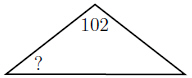6.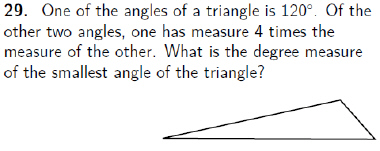7.8.

What is the value of “x”?9.

What is the value of “x”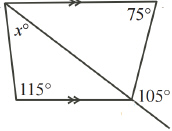10.

Suppose AD=BD=DC, and angle C is 35 degrees, what is the value of angle BAC?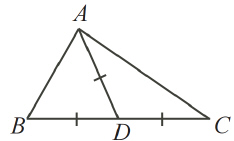11.

If the two horizontal lines are paralle, then what is the value of angle “x”?12.

What is the value of angle “y”?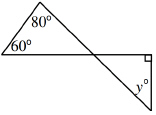13.

What is the value of “x”? Give your answer as a fraction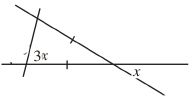14.

What is the value of “x”?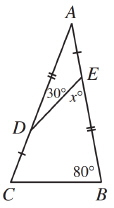15.

Find the value of “x”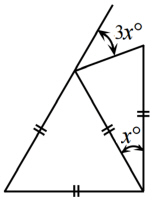16.

Find the value of “x”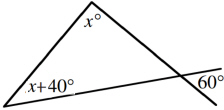17.

Find the value of the missing angle “x”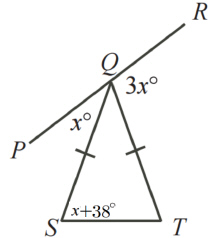18.

What is the value of “x”?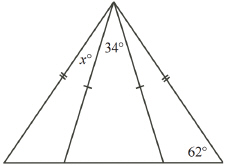19.

What is the value of “x+y” if PU=PV?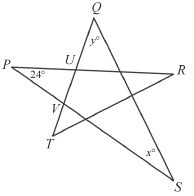20.

What is the value of angle “X”?